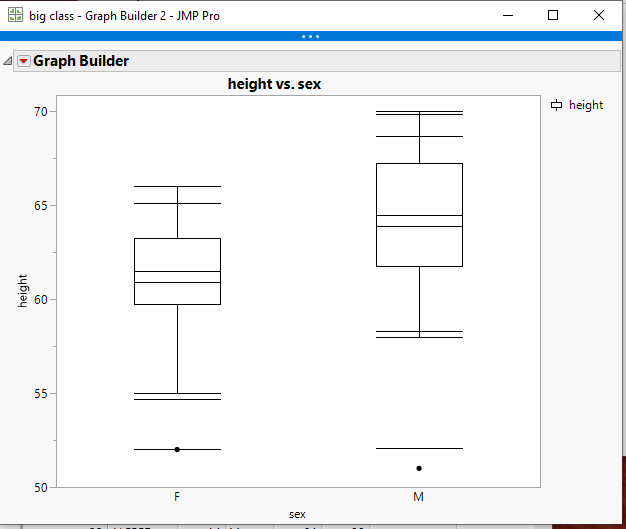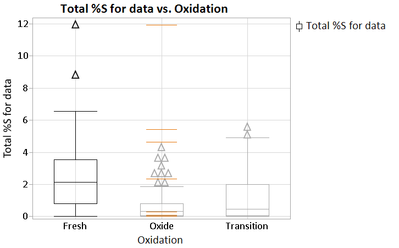Choose Language Hide Translation Bar
Highlighted

## Is there a way to display 95th, 90th 75th, 50th, 25th, 10th and 5th percentiles in box plots in graph builder or one way plots

In my work we display 95th, 90th 75th, 50th, 25th, 10th and 5th percentiles + the mean in our box plots in excel (which is painful and time consuming) – is there a way of replicating this in JMP oneway or graph builder?

andrew.botfield@geochemistry.com.au

8 REPLIES 8
Highlighted

## Re: Is there a way to display 95th, 90th 75th, 50th, 25th, 10th and 5th percentiles in box plots in graph builder or one way plots

There is not a selectable way to do this, but by adding a simple script to the output, it can be done.  Below is a script that adds in the .05,.10,mean,.90 & .95 lines.  The .25, .5 and .75 are automatically generated with the Box PlotHere is the script

``````names default to here(1);
dt=open("\$SAMPLE_DATA/big class.jmp");

// Get the statistics
Summarize(
dt,
byGroup = By( :sex ),
p05 = Quantile( :height, .05 ),
p10 = Quantile( :height, .10 ),
p90 = Quantile( :height, .90 ),
p95 = Quantile( :height, .95 ),
mean = Mean( :height )
);

gb = Graph Builder(
Size( 528, 450 ),
Show Control Panel( 0 ),
Variables( X( :sex ), Y( :height ) ),
Elements( Box Plot( X, Y, Legend( 6 ) ) )
);

statList = {"p05", "p10", "mean", "p90",
"p95"};

Report( gb )[FrameBox( 1 )] <<
Pen Color( Black );
For( s = 1, s <= N Items( statList ), s++,
myMat = Eval( Parse( statList[s] ) );
For( i = 1, i <= 2, i++,
matx = [0, 0];
matx = Matrix( i - 1 - .18 );
matx = i - 1 + .18;
maty = [0, 0];
matY = Matrix( myMat[i] );
matY = Matrix( myMat[i] );
Line( matx, maty );
);
);
);``````
Jim
Highlighted

## Re: Is there a way to display 95th, 90th 75th, 50th, 25th, 10th and 5th percentiles in box plots in graph builder or one way plots

Hi Jim - thank you so much for getting back so quickly - will give this a go and let you know - cheers!
Highlighted

## Re: Is there a way to display 95th, 90th 75th, 50th, 25th, 10th and 5th percentiles in box plots in graph builder or one way plots

Hi Jim, that is fantastic (have been playing with the script and works well. Would you be able to give me some further script clues on the following (thanks in advance):

1. How to specify different colours for different groups of stats added? (e.g. mean versus the quantile values)
2. How to change thickness of displayed lines?
3. Is there an easy way to display the newly added stats on the graph? (i.e. as captions, etc)
4. Is there an easy way to remove the outlier whiskers and replace with new stat whiskers?

Highlighted

## Re: Is there a way to display 95th, 90th 75th, 50th, 25th, 10th and 5th percentiles in box plots in graph builder or one way plots

All of your questions, except for the whiskers question are answered in the Scripting Index.

Help==>Scripting Index

If you scroll down the list of objects and functions on the left hand side, you will find a selection called Graphics.  If you click on it, it will expose all of the graphical functions that can be used.

Concerning the Whiskers, they can only be changed in color and line thickness, and the color and thickness will be for the entire box plot.

If you chose to do so, you can just use a Graph Box() and draw your own box plots.

Jim
Highlighted

## Re: The script only displays whiskers on one of the X variables - but the same script example displays for both sexes

Hi Jim,

Using the text below I only get whiskers on one of the X variables - what modifications would you make to the script below to make it display on all of X variables - also see attached - thanks in advance for your help!

``````Names Default To Here( 1 );

dt = Open( "Insitu Compiled.jmp" );

// Get the statistics
Summarize(
dt,
byGroup = By( :Oxidation ),
p05 = Quantile( :Total %S for data, .05 ),
p10 = Quantile( :Total %S for data, .10 ),
p90 = Quantile( :Total %S for data, .90 ),
p95 = Quantile( :Total %S for data, .95 ),
Mean = Mean( :Total %S for data, ),
Min = Min( :Total %S for data, ),
Max = Max( :Total %S for data, )
);

gb = Graph Builder(
Size( 528, 450 ),
Show Control Panel( 0 ),
Variables( X( :Oxidation ), Y( :Total %S for data ) ),
Elements( Box Plot( X, Y, Legend( 6 ) ) )
);

statList = {"p05", "p10", "p90", "p95", "Mean", "Min", "Max"};

Report( gb )[FrameBox( 1 )] << Add Graphics Script(
Pen Color( orange );
Pen Size( 1 );
Line Style( "Solid" );
For( s = 1, s <= N Items( statList ), s++,
myMat = Eval( Parse( statList[s] ) );
For( i = 1, i <= 2, i++,
matx = [0, 0];
matx = Matrix( i - 1 - .18 );
matx = i - 1 + .18;
maty = [0, 0];
matY = Matrix( myMat[i] );
matY = Matrix( myMat[i] );
Line( matx, maty );
);
);
);``````Highlighted

## Re: The script only displays whiskers on one of the X variables - but the same script example displays for both sexes

I figured it out – needed to delete rows at the bottom of the table and make the number 3 instead of 2 (which I assume is the number of X axis displays) – whoo!

For( i = 1, i <= 3, i++,

matx = [0, 0];

matx = Matrix( i - 1 - .18 );

matx = i - 1 + .18;

maty = [0, 0];

matY = Matrix( myMat[i] );

matY = Matrix( myMat[i] );

Line( matx, maty );

);

);

);

Highlighted

## Re: The script only displays whiskers on one of the X variables - but the same script example displays for both sexes

Change it to ......N Items(byGroup).....it will then become a generic setting

``````For( i = 1, i <= N Items( byGroup ), i++,
matx = [0, 0];
matx = Matrix( i - 1 - .18 );
matx = i - 1 + .18;
maty = [0, 0];
matY = Matrix( myMat[i] );
matY = Matrix( myMat[i] );
Line( matx, maty );
);``````

Jim
Highlighted

## Re: The script only displays whiskers on one of the X variables - but the same script example displays for both sexes

excellent - thank you!

Article Labels